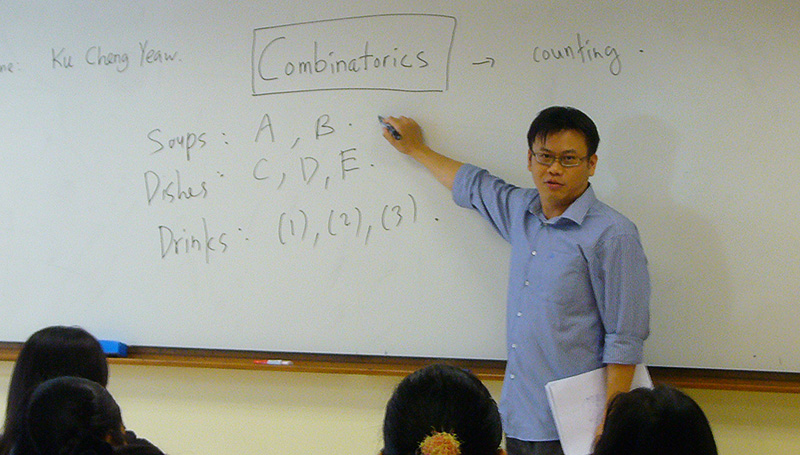### Please be informed that registration for June 2019 has closed. Thank you for your interest.This is a 5-day (15-hour) programme covering 5 topics in mathematics.

The topics include

• Number theory: Basic number theory concepts such as common multiples, unit digit, divisibility and remainder will be covered. Students will be taught pattern recognition and useful techniques to solve complex problems involving whole numbers.
• Combinatorics: This is the branch of mathematics that studies counting problems. Students will learn systematic and efficient ways to do seemingly complicated counting problems.
• Geometry: Students will be introduced geometrical figures such as polygons and circles. They will learn formulas and techniques to solve geometrical problems involving areas and angles of such figures.
• Logic and Games: Logical reasoning plays an important role in solving mathematical problems. In this session, students are required to collate information and analyse situation to solve some interesting logical puzzles.
• Arithmetic: Students will be introduced basic rules for arithmetic and some shortcuts and techniques for solving complex arithmetical problems. They will then use the techniques to solve number puzzles.